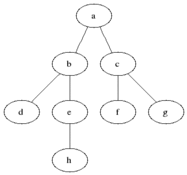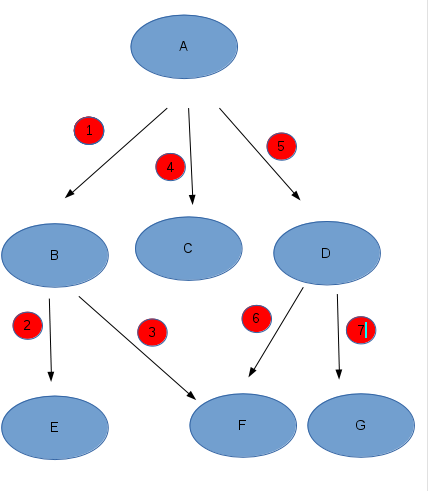# 深度优先搜索和广度优先搜索及典例分析(走迷宫问题(BFS)和棋盘问题(DFS))

### 广度优先搜索(BFS)• 具体思想：从图中某顶点a出发，在访问了a之后依次访问a的各个未曾访问过的邻接点，然后分别从这些邻接点出发依次访问它们的邻接点，并使得“先被访问的顶点的邻接点先于后被访问的顶点的邻接点被访问，直至图中所有已被访问的顶点的邻接点都被访问到。如果此时图中尚有顶点未被访问，则需要另选一个未曾被访问过的顶点作为新的起始点，重复上述过程，直至图中所有顶点都被访问到为止。

• 用途：求最短路径或最优方案
举个例子吧：
5 X 5迷宫问题
定义一个二维数组：

int maze = {

0, 1, 0, 0, 0,

0, 1, 0, 1, 0,

0, 0, 0, 0, 0,

0, 1, 1, 1, 0,

0, 0, 0, 1, 0,


};

它表示一个迷宫，其中的1表示墙壁，0表示可以走的路，只能横着走或竖着走，不能斜着走，要求编程序找出从左上角到右下角的最短路线。
Input
一个5 × 5的二维数组，表示一个迷宫。数据保证有唯一解。
Output
左上角到右下角的最短路径，格式如样例所示。
Sample Input

0 1 0 0 0
0 1 0 1 0
0 0 0 0 0
0 1 1 1 0
0 0 0 1 0

Sample Output

(0, 0)
(1, 0)
(2, 0)
(2, 1)
(2, 2)
(2, 3)
(2, 4)
(3, 4)
(4, 4)


#include<stdio.h>
#include<stdlib.h>
#include<string.h>

#define N 100
int head  ,rear ,num = 0;
typedef struct node{

int x , y ;//坐标
struct node * next ;

} node_t;
node_t  b[N],way[N] ;//队列b，和记录路的座标的结构体数组
void go_map();
void cover() ;
void init();
void print();
node_t * pop();
int a[N][N];//构造地图
int  main(){
while(1){
memset(a , 0 , sizeof(a));
int i , j ;
for( i =1 ; i< 6 ; i ++){
for( j = 1 ; j < 6; j++) {
if(scanf("%d",&a[i][j]) == EOF){//传入地图
//数据，注意这儿是从a开始存的，后面将地图边缘用1围起来
return 0;
}
}
}
cover();
init();//初始化队列
go_map();
print();
}
return 0;
}
void print(){

int i;
for(i = num-1 ; i >= 0 ; i--){//打印最短路径
printf("(%d, %d)\n",way[i].x,way[i].y);
}
}
void cover(){//将整个地图外围用1围起来，方便搜索

int i , j ;

for( i = 0 ; i< 7 ; i++){
for( j = 0 ;j < 7 ; j++){
if( i == 0 || i == 6 || j == 0 || j == 6){
a[i][j] = 1;
}
}
}
}
void init(){//初始化队列
rear = 0;
}
node_t * pop(){//弹出队列成员
node_t * p ;
return p ;
}
void record(int x ,int y ,node_t * next){//将走过的点信息加入队列，next为指向
//上一个节点数据的指针

b[rear].x = x;
b[rear].y = y;
b[rear].next =next ;
rear ++;
}
void go_map(){//遍历地图
node_t * temp ;
record(1,1,NULL);
while(1){

temp = pop();

if( temp -> x == 5 && temp->y == 5){//当走到终点时，用way 数
//组又保存返回到起点的路径

while(temp != NULL){//将第一个数据的next 指针指向空，
//作为返回起点的终止条件。
way[num].x = temp->x-1;
way[num].y =temp->y-1 ;
num ++ ;
temp = temp->next;
}
return ;
}
if(a[temp->x][temp->y - 1]!= 1)//遍历四个方向，只要满足条件，
//就加入队列
{

record(temp->x , temp->y -1 ,temp);
a[temp->x][temp->y - 1] = 1 ;

}
if(a[temp->x][temp->y + 1]!=1){

record(temp->x ,temp->y + 1 ,temp);
a[temp->x][temp->y + 1] = 1;
}
if(a[temp->x-1][temp->y]!= 1){

record(temp->x- 1 ,temp->y , temp);
a[temp->x - 1][temp->y] = 1;
}
if(a[temp->x + 1][temp->y]!= 1){
record(temp->x + 1 ,temp->y ,temp);
a[temp->x + 1][temp->y] = 1;
}
}
}

### 深度优先搜索（DFS）A -> B ->E ->F ->C ->D ->G

• 具体思想：

①访问顶点a；

②依次从a的未被访问的邻接点出发，对图进行深度优先遍历；直至图中和a有路径相通的顶点都被访问；

③若此时图中尚有顶点未被访问，则从一个未被访问的顶点出发，重新进行深度优先遍历，直到图中所有顶点均被访问过为止。

不规则棋盘问题

在一个给定形状的棋盘（形状可能是不规则的）上面摆放棋子，棋子没有区别。要求摆放时任意的两个棋子不能放在棋盘中的同一行或者同一列，请编程求解对于给定形状和大小的棋盘，摆放k个棋子的所有可行的摆放方案C。


Input

Output

Sample Input

2 1
#.
.#
4 4
...#
..#.
.#..
#...
-1 -1


Sample Output

2
1


#include<stdio.h>
#include<stdlib.h>
#include<string.h>
#define N 10
void search(int x, int y);
int columns[N];//记录第n列的状态，存在棋子且遍历过状态为1，否则为0
int num , fang_an ,area;//棋子数，方案数，行列数
char qipan[N][N];//设置字符串数组
int main(){

int j,i ;
while((scanf("%d%d",&area,&num))!=EOF){
while('\n'!=getchar());//输入完缓冲区会有换行符，后面
//要输入字符所以得清理缓冲区，本人惯用吃换行手段
if(area == -1 && num==-1 )break;
memset(qipan, 0 , sizeof(qipan));

for(i = 0; i < area ;i++  ){//输入字符串
scanf("%s",qipan[i]);
while('\n'!=getchar());
}

fang_an = 0;//将可行反案设置为0

memset(columns,0,sizeof(columns));//将记录行数的数组元素初始化0

search(0 ,0);//从坐标为(0,0)处开始走
printf("%d\n",fang_an);
}

}
void search(int x ,int y){
int i,j;

if(y == num ){//棋子数等于列数时，使方案递增，并退出当前层递归

fang_an++ ;
return ;
}
for( i = x ; i< area ; i++){

for( j = 0 ;j< area ; j++){

if((!columns[j]) && qipan[i][j] == '#'){//判断当前
//列是否已存在遍历过的棋子,并判断当前坐标中是否放的是棋子

columns[j] = 1;//若是的话，将当前列的状态改为1

search(x+1,y+1);

columns[j] = 0; //既然要退出了本层递归，就将本
//层行的状态改为初始状态
}

}
}
return ;
}


### 总结

优缺点：BFS:对于解决最短或最少问题特别有效，而且寻找深度小，但缺点是内存耗费量大（需要开大量的数组单元用来存储状态）。
DFS：对于解决遍历和求所有问题有效，对于问题搜索深度小的时候处理速度迅速，然而在深度很大的情况下效率不高

题型归类

• 坐标类型搜索 ：这种类型的搜索题目通常来说简单的比较简单，复杂的通常在边界的处理和情况的讨论方面会比较复杂，分析这类问题，我们首先要抓住题目的意思，看具体是怎么建立坐标系（特别重要），然后仔细分析到搜索的每一个阶段是如何通过条件转移到下一个阶段的。确定每一次递归（对于DFS）的回溯和深入条件，对于BFS，要注意每一次入队的条件同时注意判重。要牢牢把握目标状态是一个什么状态，在什么时候结束搜索。还有，DFS过程的参数如何设定，是带参数还是不带参数，带的话各个参数一定要保证能完全的表示一个状态，不会出现一个状态对应多个参数，而这一点对于BFS来说就稍简单些，只需要多设置些变量就可以了。

• 数值类型搜索：这种类型的搜索就需要仔细分析分析了，一般来说采用DFS，而且它的终止条件一般都是很明显的，难就难在对于过程的把握，过程的把握类似于坐标类型的搜索（判重、深入、枚举），注意这种类型的搜索通常还要用到剪枝优化，对于那些明显不符合要求的特殊状态我们一定要在之前就去掉它，否则它会像滚雪球一样越滚越大，浪费我们的时间 。
这次解题感觉很多地方并没有说清，若大神有疑问或发现一些bug，还请指正！！！

©️2019 CSDN 皮肤主题: 精致技术 设计师: CSDN官方博客